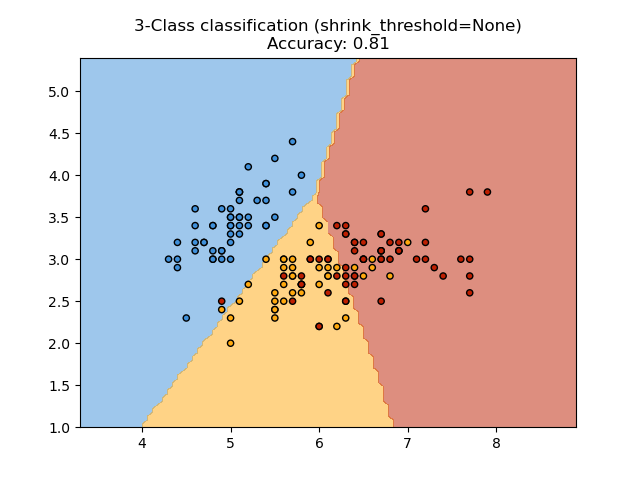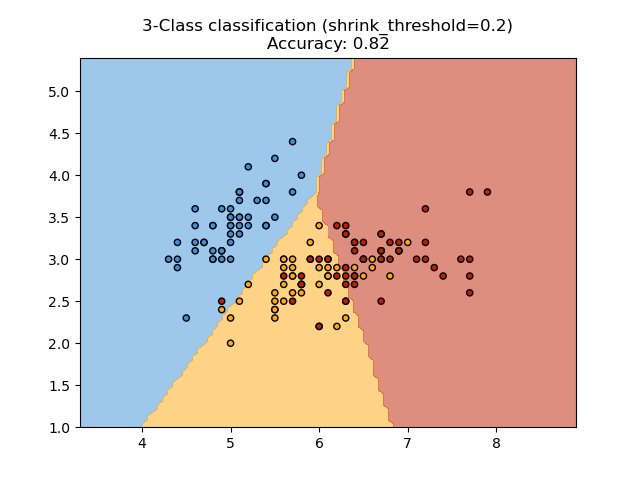# Nearest Centroid Classification¶

Sample usage of Nearest Centroid classification. It will plot the decision boundaries for each class.

••None 0.8133333333333334
0.2 0.82


import matplotlib.pyplot as plt
import numpy as np
from matplotlib.colors import ListedColormap

from sklearn import datasets
from sklearn.inspection import DecisionBoundaryDisplay
from sklearn.neighbors import NearestCentroid

# import some data to play with
# we only take the first two features. We could avoid this ugly
# slicing by using a two-dim dataset
X = iris.data[:, :2]
y = iris.target

# Create color maps
cmap_light = ListedColormap(["orange", "cyan", "cornflowerblue"])
cmap_bold = ListedColormap(["darkorange", "c", "darkblue"])

for shrinkage in [None, 0.2]:
# we create an instance of Nearest Centroid Classifier and fit the data.
clf = NearestCentroid(shrink_threshold=shrinkage)
clf.fit(X, y)
y_pred = clf.predict(X)
print(shrinkage, np.mean(y == y_pred))

_, ax = plt.subplots()
DecisionBoundaryDisplay.from_estimator(
clf, X, cmap=cmap_light, ax=ax, response_method="predict"
)

# Plot also the training points
plt.scatter(X[:, 0], X[:, 1], c=y, cmap=cmap_bold, edgecolor="k", s=20)
plt.title("3-Class classification (shrink_threshold=%r)" % shrinkage)
plt.axis("tight")

plt.show()


Total running time of the script: (0 minutes 0.153 seconds)

Gallery generated by Sphinx-Gallery